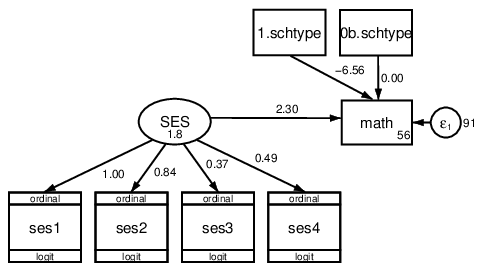# Structural Equation Modeling using Stata

## Authors

• Meghan Cain StataCorp LLC Author

## Keywords:

Structural Equation Modeling, Growth Curve Modeling, Mediation, Software, Stata

## Abstract

In this tutorial, you will learn how to fit structural equation models (SEM) using Stata software. SEMs can be fit in Stata using the sem command for standard linear SEMs, the gsem command for generalized linear SEMs, or by drawing their path diagrams in the SEM Builder. After a brief introduction to Stata, the sem command will be demonstrated through a confirmatory factor analysis model, mediation model, group analysis, and a growth curve model, and the gsem command will be demonstrated through a random-slope model and a logistic ordinal regression. Materials and datasets are provided online, allowing anyone with Stata to follow along.2021-12-02

Tutorials

## How to Cite

Structural Equation Modeling using Stata. (2021). Journal of Behavioral Data Science, 1(2), 156-177. https://doi.org/10.35566/jbds/v1n2/p7

1-10 of 20